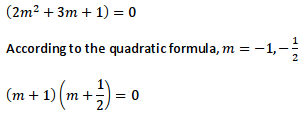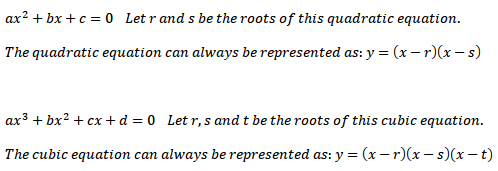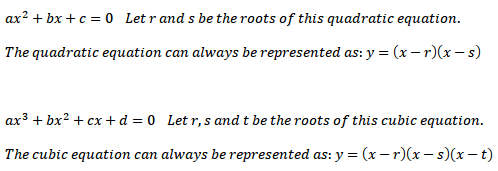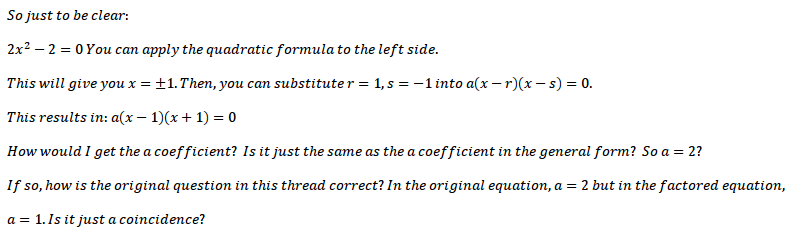# Factoring a Quadratic

ainster31

## Homework Statement

Question + attempt:## The Attempt at a Solution

Why is it that when I expand the factored form, I don't get the original equation?

Mentor

## Homework Statement

Question + attempt:## The Attempt at a Solution

Why is it that when I expand the factored form, I don't get the original equation?
You get m2 + (3/2)m + 1 = 0, which is equivalent to the original equation. Just multiply both sides by 2.

•1 person
ainster31
You get m2 + (3/2)m + 1 = 0, which is equivalent to the original equation. Just multiply both sides by 2.

Let's say we have $$2{ m }^{ 2 }+3m+1=60$$.

Would I be able to apply the quadratic formula to the left hand-side of the equation only?

Mentor
Let's say we have $$2{ m }^{ 2 }+3m+1=60$$.

Would I be able to apply the quadratic formula to the left hand-side of the equation only?
No. The Quadratic Formula requires that your equation be in the form ax2 + bx + c = 0, with a ≠ 0.

ainster31
How true is the below?Mentor
How true is the below?What do you think? These look like textbook problems. Our policy here is that we don't do the work for students. Tell me what you think and why, and I'll tell you if you're right or where you went wrong.

ainster31
What do you think? These look like textbook problems. Our policy here is that we don't do the work for students. Tell me what you think and why, and I'll tell you if you're right or where you went wrong.

They're not problems. They're statements that I wrote.

If I recall correctly, in the 10th grade, the teacher said the factored form of a quadratic equation is ##y=(x-r)(x-s)## and in the 11th grade, the teacher said the factored form of a cubic equation is ##y=(x-r)(x-s)(x-t)##. However, in the 11th grade, the teacher changed it to ##y=a(x-r)(x-s)(x-t)## later.

I don't understand why the cubic equation has the a coefficient and not the quadratic one.

Last edited:
Mentor
They're not problems. They're statements that I wrote.

If I recall correctly, in the 10th grade, the teacher said the general form of a quadratic equation is ##y=(x-r)(x-s)## and in the 11th grade, the teacher said the general form of a cubic equation is ##y=(x-r)(x-s)(x-t)##.
The general form of a quadratic function is y = ax2 + bx + c. The right side of this can be written as a(x -r)(x -s). It's possible that r and s are complex numbers, though.
However, in the 11th grade, the teacher changed it to ##y=a(x-r)(x-s)(x-t)## later.
The general form of a cubic function is y = ax3 + bx2 + cx + d. The right side can be written in factored form as a(x - r)(x - s)(x - t), with possibly some of the roots being complex. Complex roots come in pairs, so we can't have more than two complex roots in a cubic equation.

ainster31
Is there an example of a general quadratic equation where when factored, a is not 1?

Edit: Actually, never mind. Thanks.

Mentor
2m2 + 3m + 2 = 0
==> 2(m + 1)(m + 1/2) = 0

ainster31
Actually, my understanding still might be poor.Mentor
2x2 - 2 = 0
==> 2(x2 - 1) = 0
==> 2(x - 1)(x + 1) = 0

The coefficient a that appears in ax2 + bx + c = 0 is exactly the same as the one that appears in a(x - r)(x - s).

BTW, you can use the quadratic formula for this equation, but it's not necessary. After removing the common factor to get 2(x2 - 1), I just factored the expression inside the parentheses, using the formula x2 - b2 = (x - b)(x + b).

For the problem in post #1, you have
2m2 + 3m + 2 = 0
==> 2(m2 + (3/2)m + 1) = 0
==> 2(m + 1)(m + 1/2) = 0

To go from the 2nd step to the 3rd, I factored, but you can also use the quadratic formula.

Note that 2(m + 1)(m + 1/2) = 0 and (m + 1)(m + 1/2) = 0 are equivalent equations. They both have exactly the same solutions.

ainster31
Note that 2(m + 1)(m + 1/2) = 0 and (m + 1)(m + 1/2) = 0 are equivalent equations. They both have exactly the same solutions.

So what is the point of the a coefficient if you can always divide it out?

Mentor
There's not really a point. a is just the coefficient of the squared term in a quadratic equation, just like be is the coefficient of the first degree term, and c is the constant.

ainster31
Thanks for the help.

My confession is that I'm a second-year computer engineering student.

It's kind of sad. Factoring quadratic and cubic equations always bugged me. The original quadratic is from trying to solve an auxiliary equation of a differential equation. Lol

Martin Zhao
You need to multiply by 2, because when you were factoring, your formula was divided by 2.